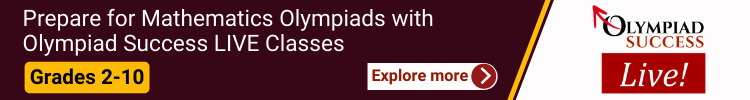All fields are required

The CREST Mathematics Olympiad is held in two levels:

Level 1: The respective school is the venue for students appearing through schools. For those students who are registered as an individual will have to take exam from home or from institute having computer with good internet connectivity.

The Level 1 exam is an objective-type test having duration of 60 minutes comprising of 35 objective-type questions for classes 1 to 4 and 50 objective-type questions for classes 5 to 10.

The exam consists of two sections:

• Section-1: Practical Mathematics
• Section-2: Achievers SectionOther Important Info:

• There are separate question papers for each and every class.
• The medium of the exam is English.
• CBSE, ICSE/ISC and other State Board syllabus is followed for the setting of test papers.
• The exam is conducted during school hours only

Level 2: The Level 2 is organised for students of classes 3 to 10 only. The qualifiers to second round would include the following:

• Top 5% of candidates in a particular class that have given the Level 1 exam. Please note that, to calculate the top 5% students, due weightage will be given to marks scored in different sections. Also, each section is accorded with a separate weightage.
• Top 25 rank holders in a particular class and in a particular zone.

If in a particular class, if a minimum of 10 students have secured 50% qualifying marks, then the respective class topper will be eligible to give the Level 2 exam.

##### Class 1

Section 1: Numerals, Number Names, Number Sense (2 digit numbers), Addition, Subtraction, Lengths, Weights and Comparisons, Time, Money, Geometrical Shapes and Solids, Patterns.

Achievers Section: Higher Order Thinking Questions - Syllabus as per Section 1

What we Offer?

• Comprehensive Test Series: 60+ worksheets + 10+ mock test papers
• Mock Test Series: 10+ mock test papers
##### Class 2

Section 1: Numerals, Number Names and Number Sense (3-Digit Numbers), Computation Operations, Length, Weight, Capacity, Time, Temperature, Money, Lines, Shapes and Solids, Pictographs, Patterns.

Achievers Section: Higher Order Thinking Questions - Syllabus as per Section 1

What we Offer?

• Comprehensive Test Series: 60+ worksheets + 10+ mock test papers
• Mock Test Series: 10+ mock test papers
##### Class 3

Section 1: Numerals, Number Names and Number Sense (4-digit numbers), Computation Operations, Fractions, Length, Weight, Capacity, Temperature, Time, Money, Geometry, Data Handling.

Achievers Section: Higher Order Thinking Questions - Syllabus as per Section 1

What we Offer?

• Comprehensive Test Series: 60+ worksheets + 10+ mock test papers
• Mock Test Series: 10+ mock test papers
##### Class 4

Section 1: Numerals and Number Names, Number Sense (5-digit numbers), Computation Operations, Fractions, Length, Weight, Capacity, Time, Money, Geometry, Perimeter of Various Shapes, Symmetry, Conversions, Data Handling.

Achievers Section: Higher Order Thinking Questions - Syllabus as per Section 1

What we Offer?

• Comprehensive Test Series: 60+ worksheets + 10+ mock test papers
• Mock Test Series: 10+ mock test papers
##### Class 5

Section 1: Numerals, Number Names and Number Sense (7 and 8 digit numbers), Computation Operations, Fractions and Decimals, Measurement of Length, Weight, Capacity, Volume, Time, Temperature and Money, Conversions, Geometrical Shapes and Solids, Angles, Perimeter of Various Shapes & Area of Rectangle and Square, Symmetry, Data Handling.

Achievers Section: Higher Order Thinking Questions - Syllabus as per Section 1

What we Offer?

• Comprehensive Test Series: 60+ worksheets + 10+ mock test papers
• Mock Test Series: 10+ mock test papers
##### Class 6

Section 1: Knowing Our Numbers, Whole Numbers, Playing with Numbers, Basic Geometrical Ideas, Understanding Elementary Shapes, Integers, Fractions, Decimals, Data Handling, Mensuration, Algebra, Ratio And Proportion, Symmetry, Practical Geometry.

Achievers Section: Higher Order Thinking Questions - Syllabus as per Section 1

What we Offer?

• Comprehensive Test Series: 60+ worksheets + 10+ mock test papers
• Mock Test Series: 10+ mock test papers
##### Class 7

Section 1: Integers, Fractions and Decimals, Exponents and Powers, Algebraic Expressions, Simple Linear Equations, Lines and Angles, Comparing Quantities, The Triangle and its Properties, Symmetry, Congruence of Triangles, Rational Numbers, Perimeter and Area, Data Handling, Visualizing Solid Shapes, Practical Geometry.

Achievers Section: Higher Order Thinking Questions - Syllabus as per Section 1

What we Offer?

• Comprehensive Test Series: 60+ worksheets + 10+ mock test papers
• Mock Test Series: 10+ mock test papers
##### Class 8

Section 1: Rational Numbers, Squares and Square Roots, Cubes and Cube Roots, Exponents and Powers, Comparing Quantities, Algebraic Expressions and Identities, Linear Equations in One Variable, Understanding Quadrilaterals, Constructions, Mensuration, Visualizing Solid Shapes, Data Handling, Direct and Inverse Variations, Factorization, Introduction to Graphs, Playing with Numbers.

Achievers Section: Higher Order Thinking Questions - Syllabus as per Section 1

What we Offer?

• Comprehensive Test Series: 60+ worksheets + 10+ mock test papers
• Mock Test Series: 10+ mock test papers
##### Class 9

Section – 1: Number Systems, Polynomials, Coordinate Geometry, Linear Equations in Two Variables, Introduction to Euclid’s Geometry, Lines and Angles, Triangles, Quadrilaterals, Areas of Parallelograms and Triangles, Circles, Constructions, Heron’s Formula, Surface Areas and Volumes, Statistics, Probability.

Achievers Section: Higher Order Thinking Questions - Syllabus as per Section 1

What we Offer?

• Comprehensive Test Series: 60+ worksheets + 10+ mock test papers
• Mock Test Series: 10+ mock test papers
##### Class 10

Section - 1:Real Numbers, Polynomials, Pair of Linear Equations in Two Variables, Quadratic Equations, Arithmetic Progressions, Triangles, Coordinate Geometry, Introduction to Trigonometry, Some Applications of Trigonometry, Circles, Constructions, Areas Related to Circles, Surface Areas and Volumes, Statistics, Probability.

Achievers Section: Higher Order Thinking Questions - Syllabus as per Section 1

What we Offer?

• Comprehensive Test Series: 60+ worksheets + 10+ mock test papers
• Mock Test Series: 10+ mock test papers